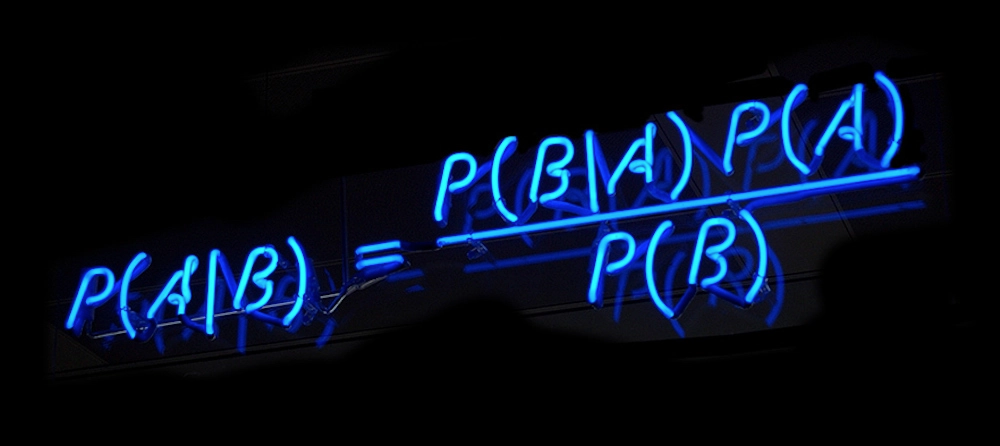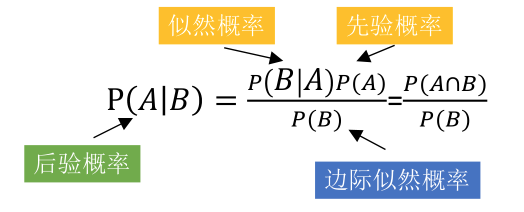2019/04/10 10:10

[toc]

# 一、贝叶斯## 什么是先验概率、似然概率、后验概率• 先验概率：男人的老婆没有任何情况，出轨的概率
• 似然概率：男人的老婆出轨了，手机里有暧昧短信的概率
• 后验概率：男人发现老婆手机有暧昧短信，计算他老婆出轨概率

• 先验概率：A厂的产品占产品总数的比例
• 似然概率：A厂的次品率
• 后验概率：已知一件产品是次品，推断这件产品来自在A厂的概率。（次品可能来自ABC中任意一个厂）

## 公式推导• $P(A|B)$：表示事件B已经发生的前提下，事件A发生的概率，叫做事件B发生下事件A的条件概率。

• $P(AB)$：表示事件A和事件B同时发生的概率

• $P(AB)=P(BA)$，可得$P(AB)=P(BA)=P(B|A)P(A)=P(A|B)P(B)$

• 通过$P(A|B)$来求$P(B|A)$，可得$P(B|A)=\frac {P(A|B)P(B)} {P(A)}$

• 分母$P(A)$可以根据全概率公式分解为：$P(A)=\sum_{i=1}^n P(B_i)P(A|B_i)$

• 最终公式变为：$P(B|A)=\frac {P(A|B)P(B)} {\sum_{i=1}^n P(B_i)P(A|B_i)}$

# 二、为什么需要朴素贝叶斯$P(X,Y)=P(Y)⋅P(X∣Y)=P(X)⋅P(Y∣X)$

• $P(Y)$：每个类别出现的概率，先验概率
• $P(X|Y)$：给定的类别下不同属性出现的概率，似然概率

# 三、朴素贝叶斯是什么朴素贝叶斯，加了个朴素，意思是更简单的贝叶斯。 朴素贝叶斯假定样本的不同属性满足条件独立性假设，并在此基础上应用贝叶斯定理执行分类任务。

## 条件独立$P(X=x∣Y=c)=$

$P(X(1)=x(1),X(2)=x(2),⋯,X(n)=x(n)∣Y=c)$

$\prod_{j=1}^nP(X^j=x^j|Y=c)$

## 举例：长肌肉## 拉普拉斯平滑## 半朴素贝叶斯0
0 收藏

### 作者的其它热门文章0 评论
0 收藏
0